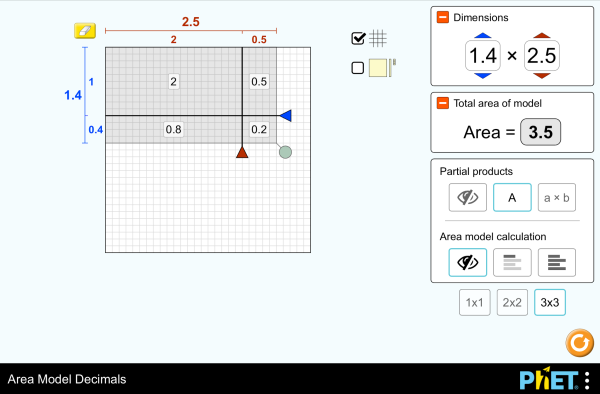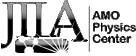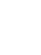# වර්ගඑල ආකෘති දශමබාගත කරන්නඔබ්බනවා වසන්න මෙම අනුහුරුකරණයෙහි ක්‍රියාත්මක පිටපතක් කාවද්දන්න. මෙම අනුහුරුකරණයෙහි ක්‍රියාත්මක පිටපතක් කාවැද්දීමට මෙම HTML භාවිත කරන්න. HTML වල ඇති ඇති"width" සහ "height" යන HTML හි ගුණාංග වෙනස් කරමින් .ඔබ කාවැද්දූ අනුහුරුකරණයෙහි පළල සහ උස වෙනස් කල හැකිය. මත ක්ලික් කරන විට අනුහුරුකරණ දියත් කරන රූපයක් කාවද්දන්න
ක්‍රියාත්මක කිරීමට ක්ලික් කරන්න
"Click to Run" වචන සමග තිර පිටපතක් පෙන්වීම සදහා මෙම HTML කේතය භාවිතා කරන්න ගුණ කිරීම Decimals Area Model PhET සහය ලබන්නේසහ ඔබවැනි අධ්‍යාපනඥයින්

### අන්තර්ගතය

• ගුණ කිරීම
• Decimals
• Area Model
• Factors
• Partial Products

### විස්තර

Build rectangles of various sizes and relate multiplication to area. Partition a rectangle into smaller areas and discover new strategies for multiplying decimals!

### ආදර්ශ ඉගෙනුම් ඉලක්ක

• Recognize that area represents the product of two numbers and is additive.
• Develop and justify a strategy that uses the area model to simplify a decimal multiplication problem.
• Devise a strategy to determine the product of two rational numbers (or an integer and a rational number) by representing the product as an area or the sum of areas.
• Use an area model to understand why multiplying a given number by a decimal less than 1 results in a product smaller than the given number.
• Interpret the product of two rational numbers using base-ten tiles

### Standards Alignment

#### Common Core - Math

5.NF.B.4
Apply and extend previous understandings of multiplication to multiply a fraction or whole number by a fraction.
5.NF.B.4a
Interpret the product (a/b) × q as a parts of a partition of q into b equal parts; equivalently, as the result of a sequence of operations a × q ÷ b. For example, use a visual fraction model to show (2/3) × 4 = 8/3, and create a story context for this equation. Do the same with (2/3) × (4/5) = 8/15. (In general, (a/b) × (c/d) = ac/bd.)
5.NF.B.4b
Find the area of a rectangle with fractional side lengths by tiling it with unit squares of the appropriate unit fraction side lengths, and show that the area is the same as would be found by multiplying the side lengths. Multiply fractional side lengths to find areas of rectangles, and represent fraction products as rectangular areas.
5.NF.B.5
Interpret multiplication as scaling (resizing), by:
5.NF.B.5a
Comparing the size of a product to the size of one factor on the basis of the size of the other factor, without performing the indicated multiplication.
5.NF.B.5b
Explaining why multiplying a given number by a fraction greater than 1 results in a product greater than the given number (recognizing multiplication by whole numbers greater than 1 as a familiar case); explaining why multiplying a given number by a fraction less than 1 results in a product smaller than the given number; and relating the principle of fraction equivalence a/b = (n × a)/(n × b) to the effect of multiplying a/b by 1.
අනුවාදය 1.2.1

### ගුරුවරුන් ඉදිරිපත් කල ක්‍රියාකාරකම්

Games Remote Lesson ideasTrish Loeblein MS
HS
UG-Intro
K-5
HW
Remote
Chemistry
Mathematics
Physics
Area Model Decimals - Grade 6Maura Dudley MS Guided Mathematics
Middle School Math Sim AlignmentAmanda McGarry MS Other Mathematics
PRIMARIA: Alineación con programas de la SEP México (2011 y 2017)Diana López MS
K-5
Discuss
Guided
HW
Demo
Lab
Chemistry
Astronomy
Physics
Mathematics
භාෂාව Downloador RunTips
Albanian All shqipModeli i Syprinës: Decimal
Basque All EuskaraAzalera-modeloa hamartarrekin
Chinese (China) All 中文 (中国)面积模型：小数
Chinese (Taiwan) All 中文 (台灣)Area Model Decimals_面積模型與小數
Dutch All NederlandsOppervlakte model decimalen
Finnish All suomiPinta-alamalli desimaaleilla
French All françaisModélisation par les aires avec décimales
Gujarati All Gujaratiઅપૂર્ણાંકમાં ક્ષેત્રફળનું મોડેલ - નિકુંજ સવાણી દ્વારા
Hindi All हिंदीनमूने का क्षेत्रफल - दाशमिक
Hungarian All magyarTerületmodell: tizedesjegyek
Italian All italianoRappresentazione grafica del prodotto di numeri decimali
Japanese All 日本語面積と式の展開（小数）
Kazakh All KazakhАуданды ондық бөлшектермен есептеу
Korean All 한국어면적 모형: 소수
Latvian All LatviešuLaukuma modeļa decimāldaļskaitļi
Mongolian All Монгол (Монгол)Талбайн Загвар Бутархайгаар
Persian All فارسیمدل مساحت اعشاری ها
Polish All polskiModel pola - ułamki dziesiętne
Portuguese (Brazil) All português (Brasil)Modelo de Área: Decimais
Russian All русскийВычисление площади с десятичными дробями
Serbian All СрпскиМатематика преко површина-Децимални бројеви
Swedish All svenskaAreamodellen och decimaltal
Tamil All Tamilமாதிரிகளின் தசமப் பரப்பளவு
Turkish All TürkçeAlan Modelinde Çarpma İşlemi (Ondalık)
Ukrainian All українськаПлощинна модель десяткових дробів
Vietnamese All Tiếng ViệtSố thập phân với mô hình diện tích
අරාබි All العربيةنموذج مساحة الأعداد العشرية
ඉංග්‍රීසි All EnglishArea Model Decimals
ග්‍රීක් All ΕλληνικάΥπολογισμός εμβαδού - Δεκαδικοί
ජර්මන් All DeutschFlächenmodell Dezimalzahlen
ඩැනිෂ් All DanskArealmodel, decimaler
බොස්නියානු All BosanskiMatematički model površina - Decimalni brojevi
ස්පැනිෂ් All españolModelo de Áreas: Decimales
ස්පැනිෂ්(පේරු) All español (Perú)Modelo de área con decimales
ස්පැනිෂ්(මෙක්සිකෝ) All español (México)Modelo de Áreas: Decimales

HTML5 sims can run on iPads and Chromebooks, as well as PC, Mac, and Linux systems.

iOS 12+ Safari

Android:
Not officially supported. If you are using the HTML5 sims on Android, we recommend using the latest version of Google Chrome.

Chromebook:
The HTML5 and Flash PhET sims are supported on all Chromebooks.
Chromebook compatible sims

Windows Systems:

Macintosh Systems:

Linux Systems:

නිර්මාණ කණ්ඩායම තෙවන පාර්ශවීය පුස්තකාල අපගේ ස්තුතිය
• Jonathan Olson (developer)
• Karina Hensberry
• Susan Miller
• Ariel Paul
• Kathy Perkins
• almond-0.2.9.js
• base64-js-1.2.0.js
• FileSaver-b8054a2.js
• font-awesome-4.5.0
• game-up-camera-1.0.0.js
• he-1.1.1.js
• himalaya-0.2.7.js
• jama-1.0.2
• jquery-2.1.0.js
• lodash-4.17.4.js
• pegjs-0.7.0.js
• seedrandom-2.4.2.js
• text-2.0.12.js
• TextEncoderLite-3c9f6f0.js